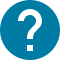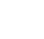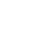大学院
HOME 大学院 Mathematics for Public Policy

UTASにアクセスできない方は、担当教員または部局教務へお問い合わせ下さい。

# Mathematics for Public Policy

Mathematical methods for public policy analysis
This is an introductory course in mathematical methods for public policy analysis, aimed at GraSPP students without strong backgrounds in mathematics. Especially, students who came from non-economics, non-engineering or non-science majors are welcomed. This course helps students develop mathematical foundation skills for applying useful mathematical techniques to public policy issues. The course consists of four parts. In the first, I introduce the foundations of differential calculus. In the second, I introduce some concepts in linear algebra. The third part is devoted to multivariate calculus and constrained static optimization. The last part provides an introduction to dynamic optimization and linear dynamic systems.

コース名

5130251
GPP-MP6Z30L3
Mathematics for Public Policy

A1 A2

2

NO

I. Single variable calculus 1. Functions and limits 2. Differential calculus 3. Applications of derivatives 4. Integral calculus (optional) II.Linear algebra 5.Linear systems 6. Matrix algebra 7. Linear independence 8. Inner product 9. Linear transformations 10. The determinant function III. Multivariable calculus 11. Partial derivative 12. Linear approximation 13. Constrained optimization IV. Dynamic analysis 14. Introduction to dynamic optimization 15. Linear dynamic systems 16. Practice using Matlab (optional)

Lecture

Your final course grade will be based on your homework (problem sets), and final exam scores. 50 percent is given for each.

No specific textbook required.

Calculus, by Gilbert Strang Linear algebra for everyone, by Gilbert Strang The ABCs of RBCs: An Introduction to Dynamic Macroeconomic Models by George McCandless

Prospective students are expected to take the screening test before the first day of the class. (Screening test problems and answers will be posted on UTAS syllabus on September 28th.) Those who score 60 percent or lower based on the self-assessment in the screening test are highly recommended to take this course. If your score is around 60 percent and you like to refresh your memory of undergraduate-level mathematics, this course could provide a good opportunity to move on to advanced courses on quantitative methods. ■■■Added on September 28th■■■ <Screening Test Problems> https://www.pp.u-tokyo.ac.jp/***** <Suggested Solutions> https://www.pp.u-tokyo.ac.jp/*****
その他
The course schedule shows the maximum coverage. Several advanced topics may not be covered depending on the pace of progress, feedback from the class, and the time spent for practice sessions using matlab. Students are encouraged to install matlab (under UTokyo license, free for students) in the class.